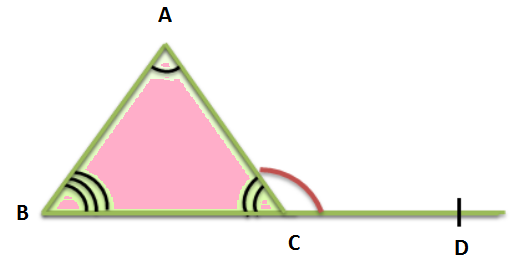# What is the exterior angle property of a triangle?

Solution:

Exterior Angle property

The exterior angle property states that if a triangle’s side gets an extension, then the resultant exterior angle would be equal to the sum of the two opposite interior angles of the triangle.According to the Exterior Angle property, the sum of measures of ∠ABC and ∠CAB would be equal to the exterior angle ∠ACD

Updated on: 10-Oct-2022

38 Views